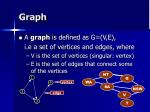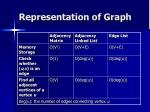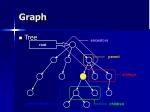Download PresentationGraph Searching

# Graph Searching - PowerPoint PPT Presentation

Graph Searching. Overview. Graph Notation and Implementation BFS (Breadth First Search) DFS (Depth First Search) Topology SCC ( Strongly Connected Component ) Centre, Radius, Diameter Multi-state BFS. What is Graph?. 1. NT. Q. 4. vertex. WA. SA. NSW. 2. edge. V. 3. T. Graph.I am the owner, or an agent authorized to act on behalf of the owner, of the copyrighted work described.
Download Presentation## Graph Searching

An Image/Link below is provided (as is) to download presentation

Download Policy: Content on the Website is provided to you AS IS for your information and personal use and may not be sold / licensed / shared on other websites without getting consent from its author.While downloading, if for some reason you are not able to download a presentation, the publisher may have deleted the file from their server.

- - - - - - - - - - - - - - - - - - - - - - - - - - E N D - - - - - - - - - - - - - - - - - - - - - - - - - -
Presentation Transcript### Graph SearchingOverview
• Graph
• Notation and Implementation
• BFS (Breadth First Search)
• DFS (Depth First Search)
• Topology
• SCC (Strongly Connected Component)
• Centre, Radius, Diameter
• Multi-state BFS### What is Graph?1

NT

Q

4

vertex

WA

SA

NSW

2

edge

V

3

T

Graph
• A graph is defined as G=(V,E),

i.e a set of vertices and edges, where

• V is the set of vertices (singular: vertex)
• E is the set of edges that connect some of the verticesGraph
• Directed/Undirected Graph
• Weighted/Unweighted Graph
• ConnectivityRepresentation of Graph
• Edge listancestors

root

parent

siblings

descendents

children

Graph
• TreeDepth-First Search (DFS)
• Strategy: Go as far as you can (if you have not visit there), otherwise, go back and try another wayImplementation

DFS (vertex u) {

mark u as visited

for each vertex v directly reachable from u

if v is unvisited

DFS (v)

}

• Initially all vertices are marked as unvisitedTopological Sort
• Topological order: A numbering of the vertices of a directed acyclic graph such that every edge from a vertex numbered i to a vertex numbered j satisfies i<j
• Topological Sort: Finding the topological order of a directed acyclic graphTsort Algorithm
• If the graph has more then one vertex that has indegree 0, add a vertice to connect to all indegree-0 vertices
• Let the indegree 0 vertice be s
• Use s as start vertice, and compute the DFS forest
• The death time of the vertices represent the reverse of topological orderExample: Assembly Line
• In a factory, there is several process. Some need to be done before others. Can you order those processes so that they can be done smoothly?
• Chris is now studying OI materials. There are many topics. He needs to master some basic topics before understanding those advanced one. Can you help him to plan a smooth study plan?Example: SCC
• A graph is strongly-connected if
• for any pair of vertices u and v, one can go from u to v and from v to u.
• Informally speaking, an SCC of a graph is a subset of vertices that
• forms a strongly-connected subgraph
• does not form a strongly-connected subgraph with the addition of any new vertexFinding Shortest Path
• How can we use DFS to find the shortest distance from a vertex to another? The distance of the first path found?• BFS tries to find the target from nearest vertices first.
• BFS makes use of a queue to store visited (but not dead) vertices, expanding the path from the earliest visited vertices.Implementation

while queue Q not empty

dequeue the first vertex u from Q

for each vertex v directly reachable from u

if v is unvisited

enqueue v to Q

mark v as visited

• Initially all vertices except the start vertex are marked as unvisited and the queue contains the start vertex only• Guarantee shortest paths for unweighted graphs
• Use queue instead of recursive functions – Avoiding stack overflowFlood Fill
• An algorithm that determines the area connected to a given node in a multi-dimensional array
• Start BFS/DFS from the given node, counting the total number of nodes visited
• Example: Squareland (HKOI 2006)Variations of BFS and DFS
• Bidirectional Search (BDS)
• Iterative Deepening Search(IDS)start

goal

Bidirectional search (BDS)
• Searches simultaneously from both the start vertex and goal vertex
• Commonly implemented as bidirectional BFSBDS Example: Bomber Man (1 Bomb)
• find the shortest path from the upper-left corner to the lower-right corner in a maze using a bomb. The bomb can destroy a wall.Bomber Man (1 Bomb)

1

2

3

4

13

12

11

12

4

10

7

6

6

7

8

9

8

5

9

5

4

3

2

1

10

11

12

13

Shortest Path length = 8

What will happen if we stop once we find a path?Iterative deepening search (IDS)
• Iteratively performs DFS with increasing depth bound
• Shortest paths are guaranteedIDS (pseudo code)

DFS (vertex u, depth d) {

mark u as visited

if (d>0)

for each vertex v directly reachable from u

if v is unvisited

DFS (v,d-1)

}

i=0

Do {

DFS(start vertex,i)

Increment iIDS
• The complexity of IDS is the same as DFSSummary of DFS, BFS
• We learned some variations of DFS and BFS
• Bidirectional search (BDS)
• Iterative deepening search (IDS)Equation
• Question: Find the number of solution of xi, given ki,pi. 1<=n<=6, 1<=xi<=150
• Vertex: possible values of
• k1x1p1 , k1x1p1 + k2x2p2 , k1x1p1 + k2x2p2 + k3x3p3 , k4x4p4 , k4x4p4 + k5x5p5 , k4x4p4 + k5x5p5 + k6x6p6